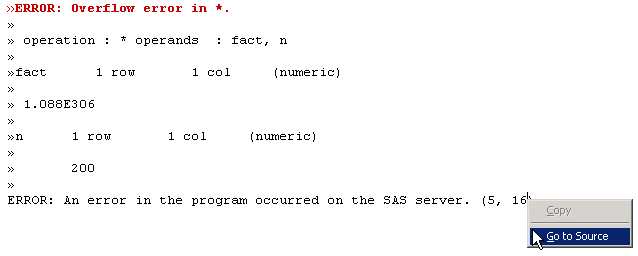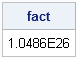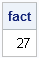NOTE: SAS stopped shipping the SAS/IML Studio interface in 2018. The references in this article to IMLPlus and SAS/IML Studio are no longer relevant.

There are three kinds of programming errors: parse-time errors, run-time errors, and logical errors. It doesn't matter what language you are using (SAS/IML, MATLAB, R, C/C++, Java,....), these errors creep up everywhere. Two of these errors cause a program to report an error, whereas the third is more insidious because the program might run to completion while silently delivering the wrong answer.

This article describes ways to find and fix each kind of error.

### Error, Errors, Everywhere

Suppose you are trying to write a SAS/IML program that computes the factorial of a number, n. The following statements might represent your initial attempt:

```proc iml; n = 200; fact = 1 do k = 1 to n; fact = fact * n; end; print fact;```

The program contains three errors—one of each kind—for an impressive error-to-line ratio of 50%. Can you find the three errors?

### Parse-Time Errors

A parse-time error occurs when the syntax of the program is incorrect. (This is also called a compile-time error for languages such as C/C++ and Java.) A parse-time error is the easiest error to correct because the parser (or compiler) tells you exactly what is wrong and on what line the problem occurs.

Common parse-time errors include mistyping a statement, forgetting a semicolon, or failing to close a set of parentheses. In strongly typed languages such as C/C++, Java, and IMLPlus, you also get a parse-time error when you try to use a variable of one type when a different type is expected. For example, it is an error to pass an integer into a function that is expecting an array or an object of a class.

In PROC IML, parse-time errors are reported in the SAS log. In SAS/IML Studio, you can select Program > Check Syntax to check your program for parse-time errors.

For the example program, SAS/IML Studio reports the following error:

```ERROR: The program contains a syntax error.
IMLPlus did not expect the following text: do (4, 1)
```

There is nothing wrong with the DO statement on Line 4, but there is a missing semicolon at the end of Line 3. As a result, the IMLPlus parser sees the statement fact = 1 do k = 1 to n; which is invalid syntax.

Fix #1: To fix the parse-time error, insert a semicolon at the end of Line 3.

### Run-Time Errors

A run-time error does not occur until the program is actually run. Common SAS/IML run-time errors include adding matrices that are different sizes, taking the logarithm of a negative value, and using the matrix index operator to specify indices that do not exist.

A previous blog post shows how to interpret error messages that appear in the SAS log when SAS/IML software encounters a run-time error.

SAS/IML Studio has some nice features for finding and fixing parse-time and run-time errors. When you run the revised test program, the SAS log reports the following error:

```»ERROR: Overflow error in *.
```

You can jump directly to the location of the error in a program window, by doing the following:

1. Right-click the error message in the Error Log window. A pop-up menu is displayed.
2. Select Go to Source.SAS/IML Studio positions the cursor in the Program window at the location of the error. By using the techniques in the previous blog post and by using the Auxiliary Input window to interrogate the value of k at the time of the error, you discover that the numerical overflow error occurs when k is 134. "Hmmmm," you think to yourself, "200! is a big number (in fact, it's 374 digits long!), perhaps I should try a more manageable value."

Fix #2: To fix the run-time error, decrease the value of n. For definiteness, set n=20.

### Logical Errors

By far, the most difficult error to find is the logical error. A program can have a logical error due to a mistyped formula or due to an incorrectly implemented algorithm. The savvy statistical programmer can use the following techniques to find and eliminate logical errors:

• Test the program on simple cases for which the result of the program is known.
• Break down the program into a sequence of basic steps and independently test each component.
• Favor clarity and simplicity when you initially write the program. After the program is working, you can profile the code and go back to optimize sections that are performance bottlenecks.

If you run the program with n=20, the program prints the following value:Is this the correct value for 20!? I don't know. Maybe I should test my program on a simpler case? I know that 3!=6, so I'll change the value of n to n=3 and re-run the program. The program prints the following value:I know that 27 isn't correct, and I recognize that 27 = 33 so I review the logic of my program statements. Sure enough, I've made a mistake. The statement inside the loop should involve the counter k, not the value n.

Fix #3: To fix the logical error, the statement inside the loop should be fact = fact * k.

### Conclusions

SAS/IML Studio contains features that help you to find and fix the three types of programming errors. You can use the techniques in this article to find errors.

However, an even better strategy is to avoid errors by "being a code samurai," which means that you should think hard about the problem and research it before you write any code. For example, if I had gone to support.sas.com and searched for "factorial," I would have discovered that SAS has a built-in FACT function, which reduces the program to a single line:

```fact = fact(20);
```

It doesn't get much simpler than that.

ShareDistinguished Researcher in Computational Statistics

Rick Wicklin, PhD, is a distinguished researcher in computational statistics at SAS and is a principal developer of SAS/IML software. His areas of expertise include computational statistics, simulation, statistical graphics, and modern methods in statistical data analysis. Rick is author of the books Statistical Programming with SAS/IML Software and Simulating Data with SAS.

1.2.•